Miscellaneous

Chapter 6 Class 12 Application of Derivatives
Serial order wise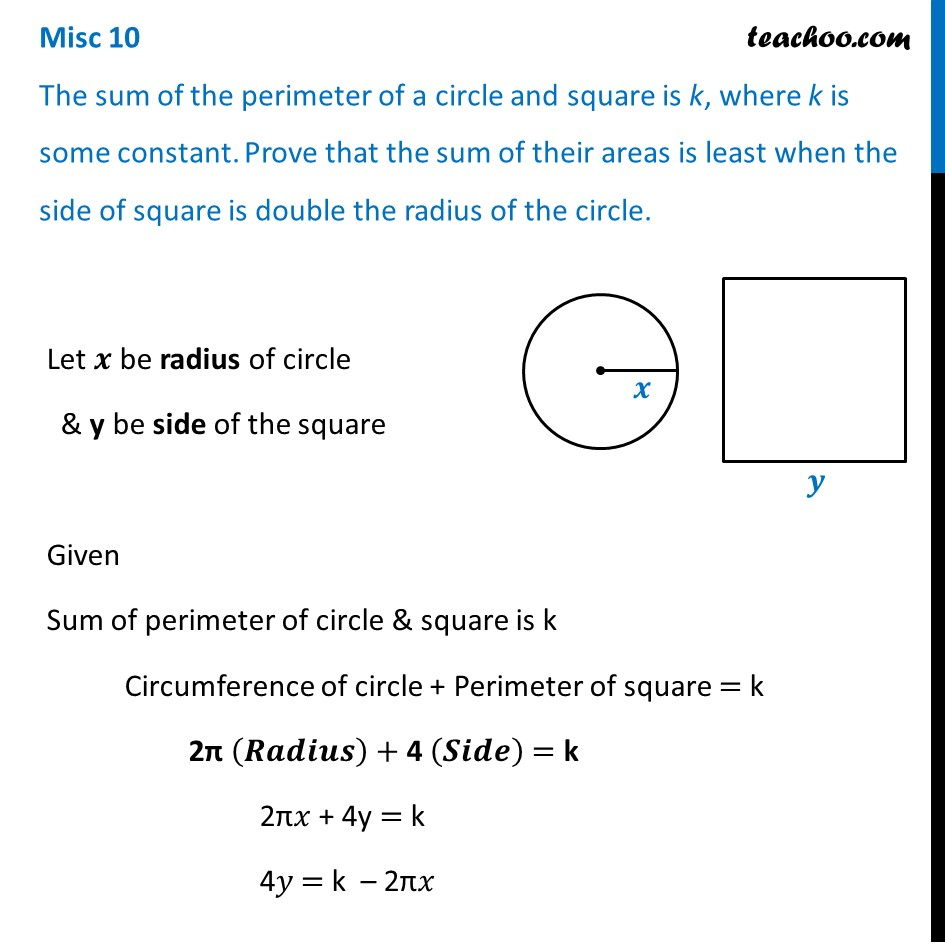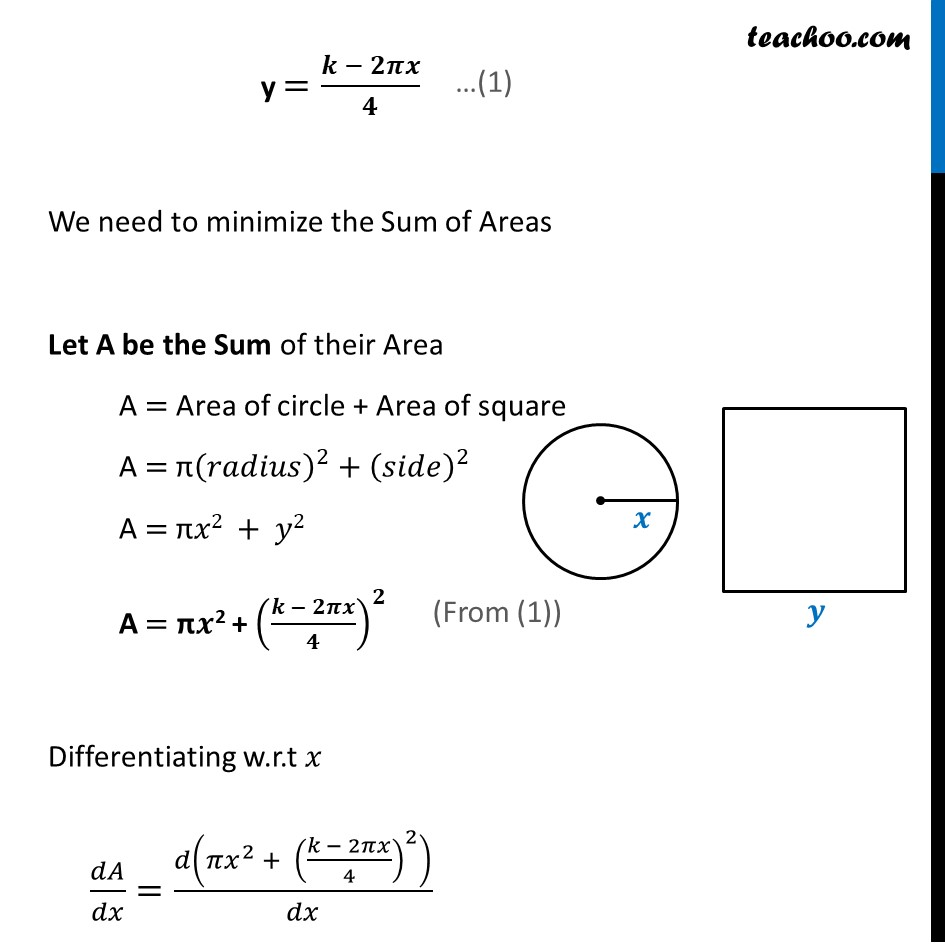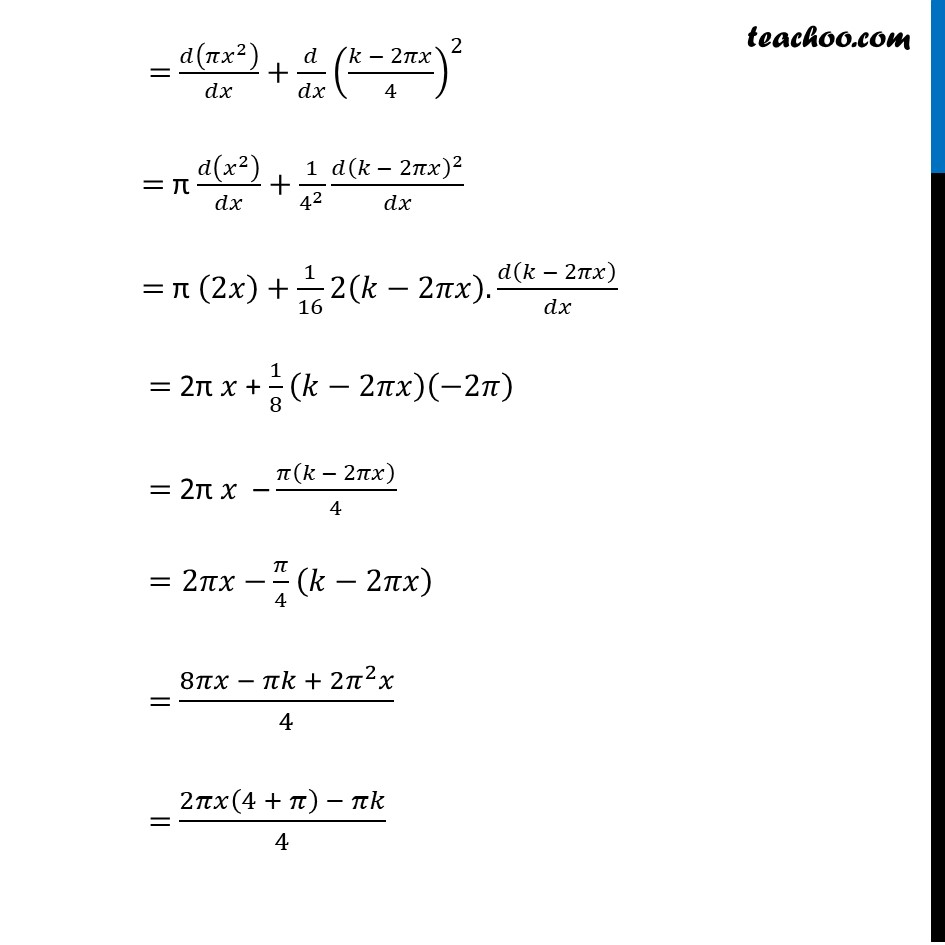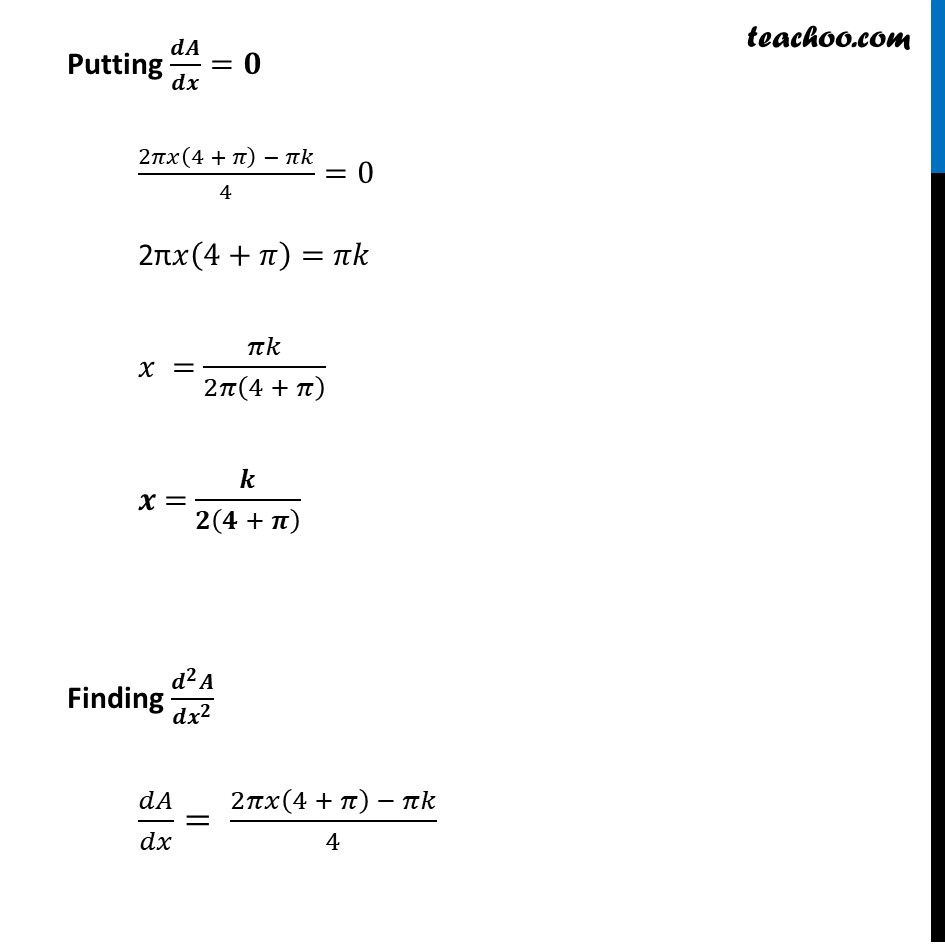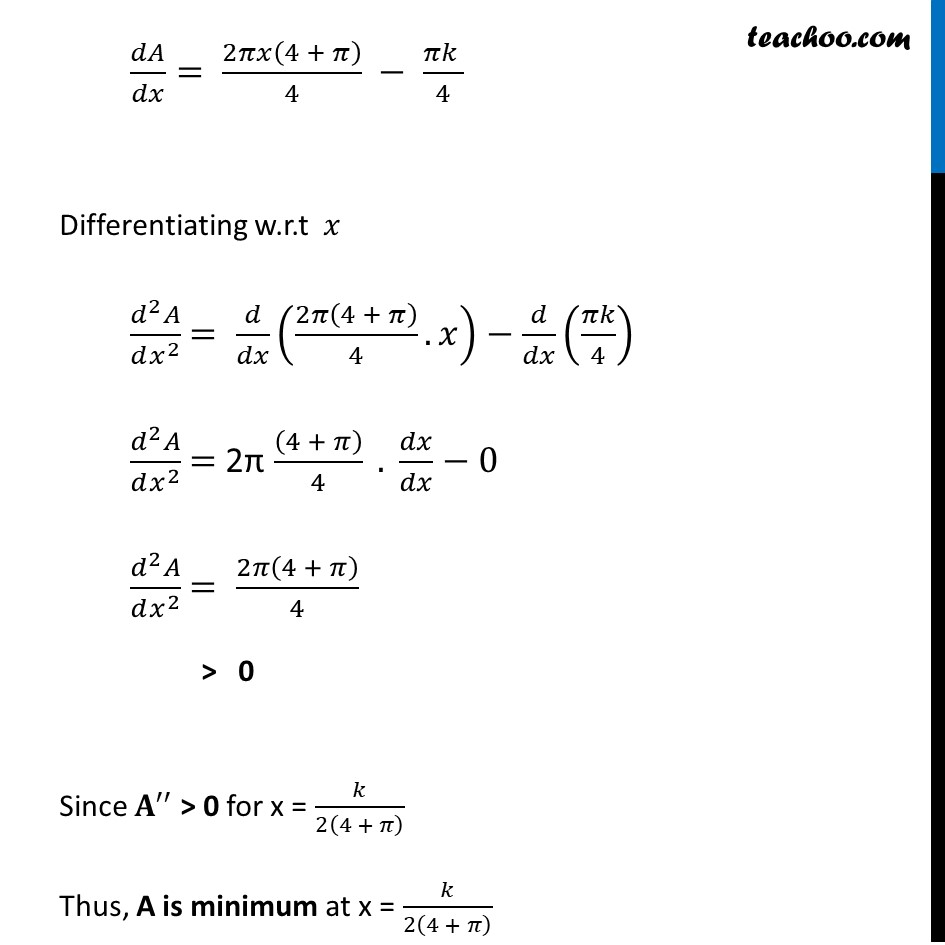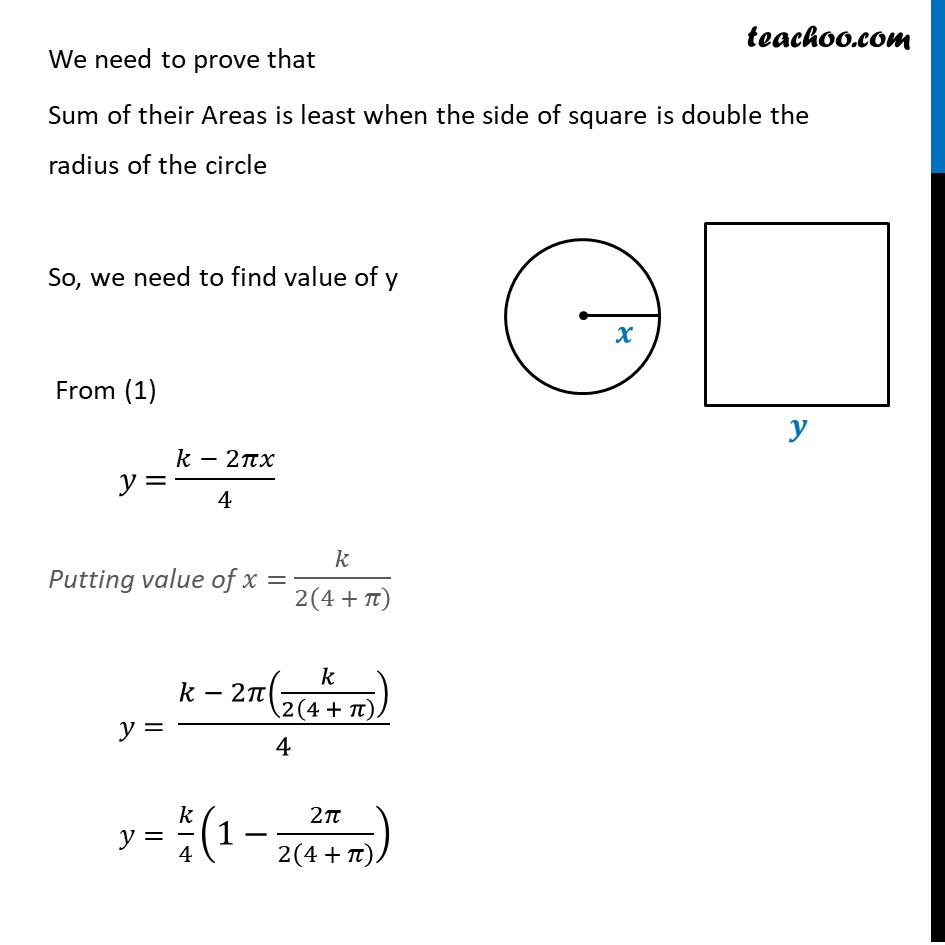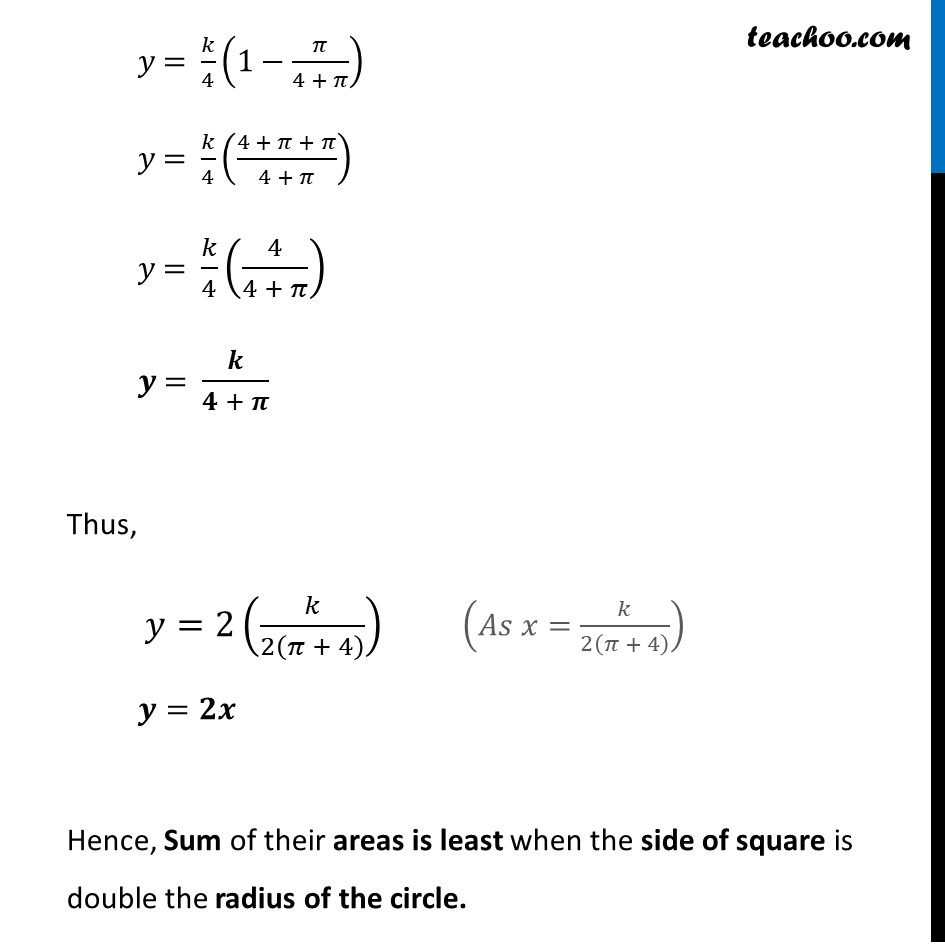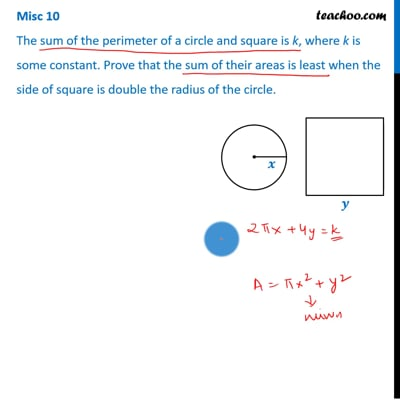This video is only available for Teachoo black users

Introducing your new favourite teacher - Teachoo Black, at only ₹83 per month

### Transcript

Misc 10 The sum of the perimeter of a circle and square is k, where k is some constant. Prove that the sum of their areas is least when the side of square is double the radius of the circle.Let 𝒙 be radius of circle & y be side of the square Given Sum of perimeter of circle & square is k Circumference of circle + Perimeter of square = k 2π (𝑹𝒂𝒅𝒊𝒖𝒔)+ 4 (𝑺𝒊𝒅𝒆) = k 2π𝑥 + 4y = k 4𝑦 = k – 2π𝑥 y =(𝒌 − 𝟐𝝅𝒙)/𝟒 We need to minimize the Sum of Areas Let A be the Sum of their Area A = Area of circle + Area of square A = π(𝑟𝑎𝑑𝑖𝑢𝑠)^2+(𝑠𝑖𝑑𝑒)^2 A = π𝑥2 + 𝑦2 A = π𝒙2 + ((𝒌 − 𝟐𝝅𝒙)/𝟒)^𝟐 Differentiating w.r.t 𝑥 𝑑𝐴/𝑑𝑥=𝑑(〖𝜋𝑥〗^2 + ((𝑘 − 2𝜋𝑥)/4)^2 )/𝑑𝑥 = 𝑑(𝜋𝑥^2 )/𝑑𝑥+𝑑/𝑑𝑥 ((𝑘 − 2𝜋𝑥)/4)^2 = π 𝑑(𝑥^2 )/𝑑𝑥+1/4^2 (𝑑(𝑘 − 2𝜋𝑥)^2)/𝑑𝑥 = π (2𝑥)+1/16 2(𝑘−2𝜋𝑥).𝑑(𝑘 − 2𝜋𝑥)/𝑑𝑥 = 2π 𝑥 + 1/8 (𝑘−2𝜋𝑥)(−2𝜋) = 2π 𝑥 – 𝜋(𝑘 − 2𝜋𝑥)/4 =2𝜋𝑥−𝜋/4 (𝑘−2𝜋𝑥) = (8𝜋𝑥 − 𝜋𝑘 + 2𝜋^2 𝑥)/4 = (2𝜋𝑥(4 + 𝜋) − 𝜋𝑘)/4 Putting 𝒅𝑨/𝒅𝒙=𝟎 (2𝜋𝑥(4 + 𝜋) − 𝜋𝑘)/4=0 2π𝑥(4+𝜋)=𝜋𝑘 𝑥 = 𝜋𝑘/2𝜋(4 + 𝜋) 𝒙 = 𝒌/𝟐(𝟒 + 𝝅) Finding (𝒅^𝟐 𝑨)/(𝒅𝒙^𝟐 ) 𝑑𝐴/𝑑𝑥= (2𝜋𝑥(4 + 𝜋) − 𝜋𝑘)/4 𝑑𝐴/𝑑𝑥= 2𝜋𝑥(4 + 𝜋)/4 − (𝜋𝑘 )/4 Differentiating w.r.t 𝑥 (𝑑^2 𝐴)/(𝑑𝑥^2 ) = 𝑑/𝑑𝑥 (2𝜋(4 + 𝜋)/4.𝑥)−𝑑/𝑑𝑥 (𝜋𝑘/4) (𝑑^2 𝐴)/(𝑑𝑥^2 ) = 2π ((4 + 𝜋))/4 . 𝑑𝑥/𝑑𝑥−0 (𝑑^2 𝐴)/(𝑑𝑥^2 ) = 2𝜋(4 + 𝜋)/4 > 0 Since 𝐀^′′ > 0 for x = 𝑘/2(4 + 𝜋) Thus, A is minimum at x = 𝑘/2(4 + 𝜋) We need to prove that Sum of their Areas is least when the side of square is double the radius of the circle So, we need to find value of y "From (1)" 𝑦 = (𝑘 − 2𝜋𝑥)/4 Putting value of 𝑥 = 𝑘/2(4 + 𝜋) 𝑦 = (𝑘 − 2𝜋(𝑘/2(4 + 𝜋) ))/4 𝑦 = 𝑘/4 (1−2𝜋/2(4 + 𝜋) ) 𝑦 = 𝑘/4 (1−𝜋/(4 + 𝜋)) 𝑦 = 𝑘/4 ((4 + 𝜋 + 𝜋)/(4 + 𝜋)) 𝑦 = 𝑘/4 (4/(4 + 𝜋)) 𝒚 = 𝒌/(𝟒 + 𝝅) Thus, 𝑦=2(𝑘/2(𝜋 + 4) ) 𝒚=𝟐𝒙 Hence, Sum of their areas is least when the side of square is double the radius of the circle.